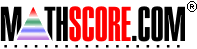Math Practice Online > free > lessons > Florida > 6th grade > Order Of Operations

## Order Of Operations

For learning about the order of operations.

 Sample Problems for Order Of Operations Lesson for Order Of Operations

### This topic aligns to the following state standards

Grade 6: Num 3. Applies order of operations when solving problems (parentheses, multiplication, division, addition, and subtraction).
Grade 7: Num 4. Applies order of operations to solve problems (parentheses, exponents, multiplication, division, addition, and subtraction).
Grade 8: Num 4. Writes and simplifies expressions from real-world situations using the order of operations.

Copyright Accurate Learning Systems Corporation 2008.